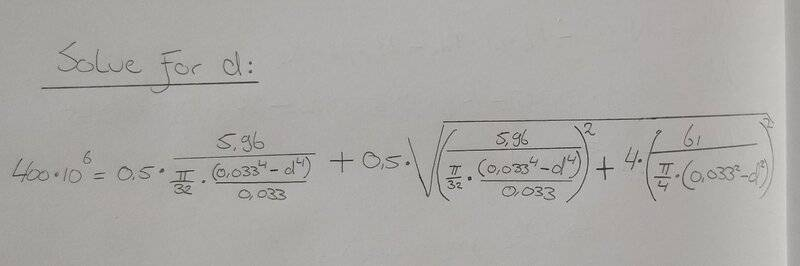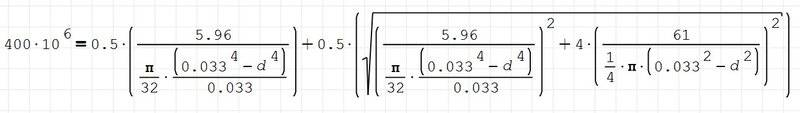# How to approach to solve this equation

• I
• Mech_LS24

#### Mech_LS24

TL;DR Summary
I would like to solve the equation for "d".
Dear mathematicians,

I am getting stuck solving this equation for "d". And what (free)software would you recommend to check this equation?Thanks a lot!

Use desmos or something or wolfram alpha

•topsquark and Mech_LS24
Summary: I would like to solve the equation for "d".

Dear mathematicians,

I am getting stuck solving this equation for "d". And what (free)software would you recommend to check this equation?
View attachment 313333

View attachment 313334

Thanks a lot!
Unless something cancels it's going to be a 10th order polynomial. And given your numbers it's highly unlikely to be factorable. W|A, Mathematica, or Matlab would be my suggestions.

-Dan

•fresh_42 and Mech_LS24
If you really want to attack that expression, I recommend the substitutions ##x=0.033^2 -d^2 ## and ##y=0.033^2+d^2##. And you should consider using a Weierstraß substitution!

•Mech_LS24, topsquark and malawi_glenn